# Typing with leftovers

Posted on 2019-10-30 by Oleg Grenrus linear

Not so long ago, an older post of mine about LLC in Agda circulated on Twitter. One comment was: it’s typing with leftovers!. What does that mean? you may ask; let me show.

This is the post with fancy LaTeX formulas :)

## #An example with times

In Church encoding of linear types post, we wrote a right rule for times as (slightly adjusted)

 Γ ==> x : X    Γ ==> y : Y
---------------------------- ⊗R
Γ₁,Γ₂ ==> pair x y : X ⊗ Y

And the fancy LaTeX version of it looks like:In this version, we somehow know how to split the context into two parts, to construct halves of times-pair.

Another way to formulate rules, is using leftover contexts:We start with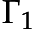context, try to construct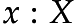. Its construction uses as much stuff from context as it needs, leaving over some context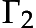. Then we continue withcontext trying to construct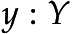, and get another residual context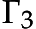. So starting withcontext, we can construct a pair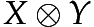, with a leftover.

## #A few more rules

We’ll need few more rules to make large enough fragment of logic to show meaningful example: Identity rule, left rule for times an right and left rules for linear implication and one. This fragment is called Multiplicative Intuitionistic Linear Logic, or MILL for short.## #Linear curry

Let us see how this work in practice. Let’s write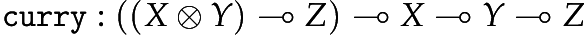.

First we’ll write in "a traditional style". I’ll omit proof terms for brevity.The proof term is

curry = \f x y -> f (pair x y)

Let’s see the with leftovers derivation tree.Not so surpsingly, the proof term is the same

curry = \f x y -> f (pair x y)

## #What’s the point?

The leftover approach seems more complicated, why to use it? There is at least two reasons. First is that proof search is a lot more direct. Let us examine the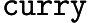derivation more closely.

This fragment of linear logic is so well behaving, that a "try whatever fits" strategy will find a solution. Let us however make it a bit more precise:

1. Use Id-rule if it applies.

2. Try right rules.

3. Try left rules.

4. Go to 1.

Let’s see how that strategy works on. First we try right rules while they match. We arrive at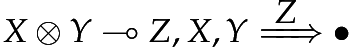situation.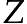is atomic formula, so no right rule match. Id doesn’t apply either. So we have to use some left rule. In this situation we don’t have a choice, as only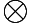L applies. The goal state has two obligations, note how we don’t yet know whatwill become.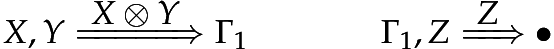We continue with the leftmost derivation.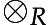rule applies. Note, how rule output and input context form kind of a chain: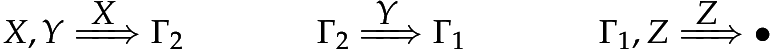Now, Id-rule applies, and we infer that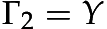.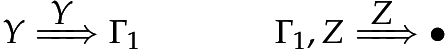Id-rule applies again,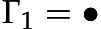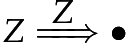and again. Finally we are left with no obligations and an empty context, as was required. Proof search completes successfully. Note how we didn’t need to an arbitrary guess how to split the context. With "traditional" sequents, we’d need to guess how to split context when using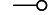L andR rules, for example in a situation like:What shouldandbe? With leftovers approach, we just continue with full context, and see what’s left.

It’s worth pointing out, that in ordinary lambda calculus, there are no such problem. If we would need to find a term for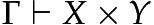, we’d split the search into to sub-searches: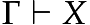and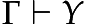, as we can reuse assumptions as many times as needed.

### #DILL and annotations

In a recent post I described DILL. It works with leftover typing as well. For example aR-rule would look like:Yet, it’s more interesting to use annotations. The assumptions stay always the same, but their usage changes:where, type type of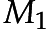,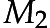and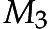depend on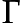(they are "lists" of the same length). Not a big change, but that’s how LLC in Agda is encoded. And I think that encoding is quite simple.

For example, in a following simple derivation the variables stay always the same: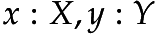, only their multiplicities change during the course. I’ll write multiplicities reusing variable names:This approach is also how you could represent and type-check linear logic using bound-library. See an example from my HaskellX 2018 talk.

So the implementation concerns are second reason to use leftover typing. This idea is described in Typing with Leftovers - A mechanization of Intuitionistic Multiplicative-Additive Linear Logic by Guillaume Allais (TYPES 2017).

### #Scoping...

We must be careful, as using leftovers scoping is different. Look closely at left over rules for. Consider the re-associating term, from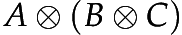to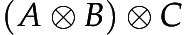, first omitting the proof terms:If I haven’t made any mistake during type-setting, that tree uses the specifiedleftover rules. But look at the resulting proof term:

case abc of
pair a bc -> pair
(pair a (case bc of
pair b c -> b))
c

Looks like c is out-of-scope, but it isn’t, given the rules. This might affect what we can do with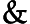and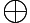rules. There are also might be some consequences for substitution. I’m not sure.

Note however, that this unconvential behaviour isn’t possible in "LLC in Agda" like encoding: there the scoping is normal, only usages are tracked with leftovers. So the above example would be rejected by a type-checked with "Variable not in scope: c" error.

## #You don’t expect this, but: regular expressions

Linear logic is a substructural logic, where so called structural rules of contraction (duplication) and weakening (removing) are removed. There’s third structural rule: exchange (using out-of-order).

• all rules: intuitionistic logic

• without contraction: affine logic

• without weakening: relevant logic

• without contraction and weakening: linear logic

• without contraction, weakening, and exchange: ordered logic

Ordered logic (also known as non-commutative linear logic, I use NCILL for intuitionistic variant) feels very restrictive, and it is. In ordered logic, "traditional"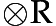rule must be read literally, assumptions have to be used in order, first, then. In intuitionistic logic context is like a set, in linear logic like a multiset, and in ordered logic like a list.This makes proof search even more simpler, you’ll ever need to look at the front of the context (when applying right rules).

And it turns out, that NCILL describes how regular expressions match: the match string forms a context (of atomic "propositions"), the regular expression is a type of expression we want, and for complete match we expect leftover context to be empty. For complete regular expressions, we’ll need to add lists to the language, but it works out.

Let’s match a string XY against X(Y|Z) regular expression, in other words is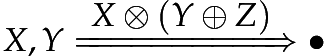derivable? It is:The proof search algorithm grew out of regular expression matching algorithm using Brzozowski derivatives. The result is in implementation of Regular expressions of types i.e. kleene-type (haddocks).

See [Certified Proof Search for Intuitionistic Linear Logic](https://gallais.github.io/proof-search-ILLWiL/). I’m rediscovering things Guillaume Allais alrady did.

## #Conclusion

What could I say. Everything seems to be connected in theoretical computer science. Linear logic and regular expressions. Lambda calculi and automata.

More seriously. We can formulate logics differently, so their expressive power stays the same, but the resulting "programming language" would look different. Maybe more convinient to use, or better in some other way.

Site proudly generated by Hakyll